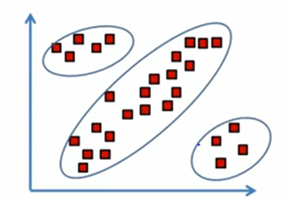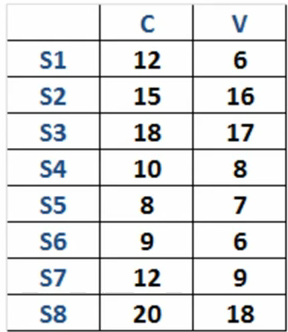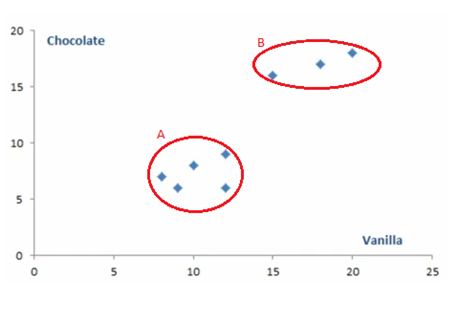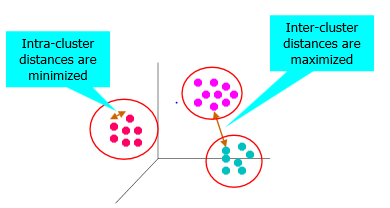## Monday, May 6, 2013

### Cluster Analysis

Cluster analysis or clustering is the task of grouping a set of objects in such a way that objects in the same group (called cluster) are more similar (in some sense or another) to each other than to those in other groups (clusters). It is a main task of exploratory data mining, and a common technique for statistical data analysis used in many fields, including machine learning, pattern recognition, imageanalysis, information retrieval, and bioinformatics.
The purpose of cluster analysis is to place objects into groups, or clusters, suggested by the data, not defined by a priori, such that objects in a given cluster tend to be similar to each other in some sense, and objects in different clusters tend to be dissimilar. You can also use cluster analysis to summarize data rather than to find "natural" or "real" clusters; this use of clustering is sometimes called dissection.As an Analyst one always faces this question, where you need to organize the data that you are observing into some kind of meaningful structure or pattern. This is where clustering comes in handy. In more simple words, clustering allows you to break the population into smaller groups where each observation within every group is more similar to each other than it is to an observation in another group. So, the idea is to group together similar kind of objects into smaller groups.

How Clustering Works
Let us take an example to understand how clustering works exactly. Imagine that we own a chain of ice cream shops. We have got a number of ice cream shops right across the country, say we have got eight of them and we sell two flavors of ice creams. We sell chocolate ice cream and vanilla ice cream. Consider the table below: There we can see the sales of both chocolate and vanilla ice cream across our eight stores. The units and time-frame are not important in this example, just imagine that this is the data that you are looking at. Now, there are many different ways one can make sense of this data. We can locate some statistics, calculate mean, median, dispersion etc. But, one very intuitive way to do this is to plot this data on a graph.Below is the graph that we get once we plot the above data on a graph:Here we plotted the sales of vanilla and chocolate ice cream for each of the eight stores. Each of the dot above represents a store. On the Y-axis we have chocolate sales and on the X-axis we have vanilla sales. Looking at the graph above it is clear that we have divided our data into two distinct groups. One group (A) has five stores and the other (B) has three stores. Obviously, the difference between two groups is with respect to the magnitude of sales. This is exactly how clustering works. It is a simple two dimensional example of how clustering works. This was an example where we had two flavors of ice cream and only eight stores. Now imagine that we have expanded our chain of ice-cream stores and instead of two flavors we are selling thirty different flavors. How this information can be plotted in a graph now? It is impossible to draw a thirty dimensional graph. What if we grow to five hundred stores? Instead of eight points on the graph we will have five hundred different points! What if there is something else which has got a million records then it will have million different points! There is a mathematical way to deal with these problems and that is what Cluster Analysis does.

What is Good Clustering?
A good clustering method will produce high quality clusters where:

·         The intra-class similarity (that is within a cluster) is high
·         The inter class similarity (that is between cluster) is low.

The second thing which describes a good clustering is its ability to discover some or all of the hidden patterns in the data.Clustering Algorithms
There are broadly three types of clustering algorithms:

·         K-means and its variants
·         Hierarchical Clustering
·         Density-based clustering

K-means clustering:
K-Means is a rather simple but well known algorithms for grouping objects, clustering. Again all objects need to be represented as a set of numerical features. In addition the user has to specify the number of groups (referred to as k) he wishes to identify.
Each object can be thought of as being represented by some feature vector in an n dimensional space, n being the number of all features used to describe the objects to cluster. The algorithm then randomly chooses k points in that vector space, these point serve as the initial centers of the clusters. Afterwards all objects are each assigned to center they are closest to. Usually the distance measure is chosen by the user and determined by the learning task.
After that, for each cluster a new center is computed by averaging the feature vectors of all objects assigned to it. The process of assigning objects and re-computing centers is repeated until the process converges. The algorithm can be proven to converge after a finite number of iterations.

Limitations of K-Means
K-mean has a problem when clusters are of differing sizes, densities or non-globular shapes. K-mean also has a problem when data contains outliers. K-mean algorithm is sensitive to outliers. It is because Centroid is average of cluster members and outlier can dominate average computation. However, these limitations can be overcome by deploying various techniques.

Hierarchical Clustering:
Hierarchical clustering produces a set of nested clusters organized as a hierarchical tree and visualized as a dendrogram. In Hierarchical clustering one does not have to assume any particular number of clusters. Any number of clusters can be obtained by cutting the dendogram at the proper level. There are two main types of hierarchical clustering: 
Agglomerative: Start with one, all inclusive cluster and at each step, mergre the closest pair of clusters until on one cluster (or K clusters) left.
Divisive: Start with one, all inclusive cluster and at each step, split a cluster until each cluster contains a single object (or there are K clusters).

Limitations and Problems with Hierarchical Clustering
The biggest problem is that, once a decision is made to combine two clusters, it cannot be undone. Also, no objective function is directly minimized. Depending on the scheme that is being deployed one or more of the following problems may arise:
·         Sensitivity to noise and outliers
·         Difficulty handling different sized clusters and complex shapes
·         Breaking large clusters

Density Based Clustering
Density based clustering algorithm has played a vital role in finding non-linear shapes structure based on the density. Density-Based Spatial Clustering of Applications with Noise (DBSCAN) is most widely used density based algorithm. It uses the concept of density reachability and density connectivity.
The advantages of using DBSCAN are:
·         It does not require a prior specification of number of clusters.
·         It is able to identify noise data while clustering
·         DBSCAN algorithm is able to find arbitrarily size and arbitrarily shaped clusters.

Limitations of Density Based Clustering
Basically there are two problems associated with Density based clustering:
·         DBSCAN algorithm fails in case of varying density clusters
·         It fails in case of neck type data-set.

Possible applications of Clustering
Clustering algorithms can be applied in many fields, for instance:
• Marketing: finding groups of customers with similar behavior given a large database of customer data containing their properties and past buying records;
• Biology: classification of plants and animals given their features;
• Libraries: book ordering;
• Insurance: identifying groups of motor insurance policy holders with a high average claim cost; identifying frauds;
• City-planning: identifying groups of houses according to their house type, value and geographical location;
• Earthquake studies: clustering observed earthquake epicenters to identify dangerous zones;
• WWW: document classification; clustering weblog data to discover groups of similar access patterns.

Requirements
The main requirements that a clustering algorithm should satisfy are:
• scalability;
• dealing with different types of attributes;
• discovering clusters with arbitrary shape;
• minimal requirements for domain knowledge to determine input parameters;
• ability to deal with noise and outliers;
• insensitivity to order of input records;
• high dimensionality;
• Interpretability and usability.

Common Problems with Clustering
There are a number of problems with clustering. Some among them are:
• current clustering techniques do not address all the requirements adequately (and concurrently);
• dealing with large number of dimensions and large number of data items can be problematic because of time complexity;
• the effectiveness of the method depends on the definition of “distance” (for distance-based clustering);
• if an obvious distance measure doesn’t exist we must “define” it, which is not always easy, especially in multi-dimensional spaces;
• The result of the clustering algorithm (that in many cases can be arbitrary itself) can be interpreted in different ways. 

References: